Electron.n. J. Diff. Eqns., Vol. 1999(1999), No. 13, pp. 1-27.

### Asymptotic properties of the magnetic integrated density of states G. D. Raikov

Abstract:
This article could be regarded as a supplement to  where we considered the Schrodinger operator with constant magnetic field and decaying electric potential, and studied the asymptotic behaviour of the discrete spectrum as the coupling constant of the magnetic field tends to infinity. To describe this behaviour when the kernel of the magnetic field is not trivial, we introduced a measure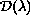defined on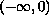called the magnetic integrated density of states''. In this article, we study the asymptotic behaviour of this measure as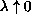and as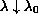,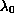being the lower bound of the support of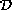.

Submitted October 11, 1998. Published April 26, 1999.
Math Subject Classification: 35J10, 35P20, 81Q10.
Key Words: magnetic Schrodinger operator, integrated density of states, spectral asymptotics.

Show me the PDF file (255K), TEX file, and other files for this article.

Georgi D. Raikov
Section of Mathematical Physics
Institute of Mathematics and Informatics